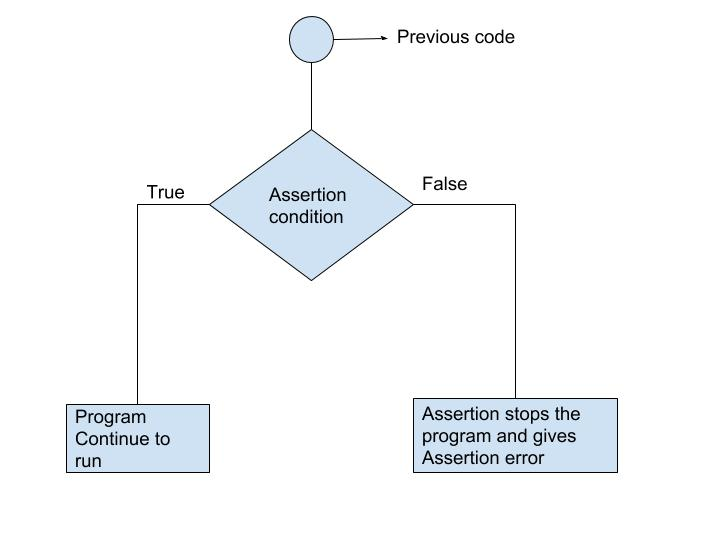# Python Assert Statement

## What is Assertion?

Assertions are statements that assert or state a fact confidently in your program. For example, while writing a division function, you're confident the divisor shouldn't be zero, you assert divisor is not equal to zero.

Assertions are simply boolean expressions that check if the conditions return true or not. If it is true, the program does nothing and move to the next line of code. However, if it's false, the program stops and throws an error.

It is also a debugging tool as it brings the program on halt as soon as any error is occurred and shows on which point of the program error has occurred.

We can be clear by looking at the flowchart below:## Python assert Statement

Python has built-in `assert` statement to use assertion condition in the program. `assert` statement has a condition or expression which is supposed to be always true. If the condition is false assert halts the program and gives an `AssertionError`.

#### Syntax for using Assert in Pyhton:

`assert <condition>`
`assert <condition>,<error message>`

In Python we can use `assert` statement in two ways as mentioned above.

1. `assert` statement has a condition and if the condition is not satisfied the program will stop and give `AssertionError`.
2. `assert` statement can also have a condition and a optional error message. If the condition is not satisfied assert stops the program and gives `AssertionError` along with the error message.

Let's take an example, where we have a function which will calculate the average of the values passed by the user and the value should not be an empty list. We will use `assert` statement to check the parameter and if the length is of the passed list is zero, program halts.

### Example 1: Using assert without Error Message

``````def avg(marks):
assert len(marks) != 0
return sum(marks)/len(marks)

mark1 = []
print("Average of mark1:",avg(mark1))``````

When we run the above program, the output will be:

`AssertionError`

We got an error as we passed an empty list mark1 to `assert` statement, the condition became false and assert stops the program and give `AssertionError`.

Now let's pass another list which will satisfy the `assert` condition and see what will be our output.

### Example 2: Using assert with error message

``````def avg(marks):
assert len(marks) != 0,"List is empty."
return sum(marks)/len(marks)

mark2 = [55,88,78,90,79]
print("Average of mark2:",avg(mark2))

mark1 = []
print("Average of mark1:",avg(mark1))``````

When we run the above program, the output will be:

```Average of mark2: 78.0
AssertionError: List is empty.```

We passed a non-empty list mark2 and also an empty list mark1 to the `avg()` function and we got output for mark2 list but after that we got an error `AssertionError: List is empty`. The `assert` condition was satisfied by the mark2 list and program to continue to run. However, mark1 doesn't satisfy the condition and gives an `AssertionError`.

## Key Points to Remember

• Assertions are the condition or boolean expression which are always supposed to be true in the code.
• `assert` statement takes an expression and optional message.
• `assert` statement is used to check types, values of argument and the output of the function.
• `assert` statement is used as debugging tool as it halts the program at the point where an error occurs.